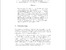# The Metric Dimension of Graph with Pendant Edges

Iswadi, Hazrul and Baskoro, Edy Tri and Simanjuntak, Rinovia and Salman, A.N.M (2008) The Metric Dimension of Graph with Pendant Edges. The Journal of Combinatorial Mathematics and Combinatorial Computing, 65. pp. 139-145. ISSN 0835-3026Preview PDF hazrul_The Metric Dimension of Graph with Pendant Edges_2008.pdf Download (151kB) | Preview

## Abstract

For an ordered set W = {w_1,w_2,...,w_k} of vertices and a vertex v in a connected graph G, the representation of v with respect to W is the ordered k-tuple r(v|W) = (d(v,w_1), d(v,w_2),..., d(v,w_k)) where d(x,y) represents the distance between the vertices x and y. The set W is called a resolving set for G if every two vertices of G have distinct representations. A resolving set containing a minimum number of vertices is called a basis for G. The dimension of G, denoted by dim(G), is the number of vertices in a basis of G. In this paper, we determine the dimensions of some corona graphs G⊙K_1, and G⊙K_m for any graph G and m ≥ 2, and a graph with pendant edges more general than corona graphs G⊙K_m.

Item Type: Article Resolving sets, metric dimension, corona graph Q Science > QA Mathematics Academic Department > Department of Mathematics and Natural Science Hazrul Iswadi 6179 08 Mar 2012 07:57 20 Mar 2012 01:38 http://repository.ubaya.ac.id/id/eprint/171

### Available Versions of this ItemView Item